# Initial Value in Calculus: Definition, Method & Example

Lesson Transcript
Instructor
Yuanxin (Amy) Yang Alcocer

Amy has a master's degree in secondary education and has taught math at a public charter high school.

Expert Contributor
Simona Hodis

Simona received her PhD in Applied Mathematics in 2010 and is a college professor teaching undergraduate mathematics courses.

In this lesson, you'lll learn about how the initial value of calculus is related to the integration process. You will see how crucial having an initial condition is and how useful it is in solving your problems. Updated: 04/22/2020

## What Is Initial Value?

Initial value in calculus is a type of problem involving the use of an initial condition. This type of problem produces an unknown constant that requires the use of an initial condition or known point to solve. When you are given an initial condition, it will look like this: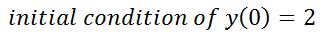This tells you that when x = 0, your y = 2. The initial condition does not have to be when x = 0. It can be any point. It's called an initial condition because it is the first bit of information you know about the newly integrated function. You need this information to fully integrate functions. Let's see why.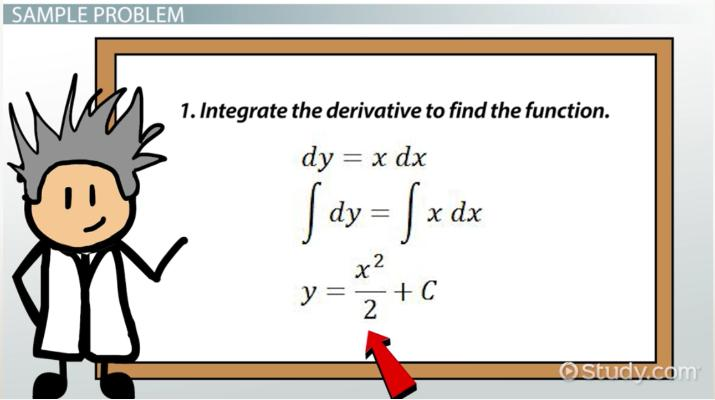An error occurred trying to load this video.

Try refreshing the page, or contact customer support.

Coming up next: Midpoint Rule: Formula & Example

### You're on a roll. Keep up the good work!

Replay
Your next lesson will play in 10 seconds
• 0:02 What Is Initial Value?
• 0:41 The Constant of Integration
• 0:57 The Method
• 1:15 Sample Problem
• 2:49 Lesson Summary
Save Save

Want to watch this again later?

Timeline
Autoplay
Autoplay
Speed Speed

## The Constant of Integration

When you calculate the indefinite integral, you end up with something called the constant of integration. It looks like this when you write it out.Because you have this unknown constant, you need a known point to plug into your equation to figure it out. This known point is your initial condition.

## The Method

The method to solving initial value problems requires just a couple of steps:

1. Integrate the derivative to find the function.

Let's see how these two steps play out with a sample problem.

## Sample Problem

The integral is also called the antiderivative because if you take the derivative of the answer, you would get the function you just integrated. With initial value problems, you're often given the derivative of the function you are trying to find along with an initial condition.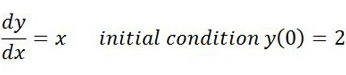To unlock this lesson you must be a Study.com Member.

### Initial Value when Acceleration Function if Given

If we know the acceleration of a moving particle, as a function of time, we can determine the position function, but we need to know two initial conditions to obtain the unique position function.

Because velocity is an antiderivative of acceleration, we integrate the acceleration function to obtain a velocity function. Integrating the velocity function, we obtain a position function.

With each integration, we have a different constant of integration.

If the acceleration is given as a(t) , then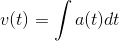and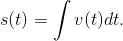When we integrate the acceleration function, we obtain a velocity function that contains a constant of integration, which is determined by using the velocity at a given point in time.

Integrating the velocity function, we obtain a position function that contains a constant of integration, which is determined uniquely by knowing the position's initial value.

### Practice Problem

Find the velocity and position functions of a particle whose acceleration is a(t)=6t if the particle starts from rest and initially the position is 2 m from the origin.

### Solution

The velocity is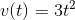because the particle starts from rest, v(0)=0 and position is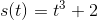because, initially s(0)=2.

### Register to view this lesson

Are you a student or a teacher?